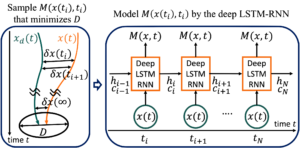# Neural Contraction Metrics (NCMs) for Robust Estimation and Control

We created a brand-new Website for this topic!Illustration of the NCM: M(x,t) denotes the optimal contraction metric; x(t) and xd(t) denote perturbed and unperturbed system trajectories; hi and ci denote the hidden states of the deep LSTM-RNN, respectively.

We have developed new deep learning-based framework for robust nonlinear estimation and control using the concept of an NCM.

NCM-based State Estimation and Control
• It globally models optimal contraction metrics sampled offline using an LSTM-RNN as depicted in (2) of the figure above (Note that the existence of such metrics is a necessary and sufficient condition for exponential stability of nonlinear systems)
• The metrics are sampled by the CV-STEM, and thus optimal in a sense that they minimize an upper bound of the steady-state Euclidean distance between perturbed and unperturbed system trajectories (see (1) of the figure above)
• The NCM is then exploited (as in the CV-STEM control) to design a real-time optimal estimator and controller for nonlinear systems with bounded disturbances utilizing their duality.

The performance of this framework is illustrated through Lorenz oscillator state estimation and spacecraft optimal motion planning problems. This work is available at https://arxiv.org/abs/2006.04361.

We have a new paper on a Neural Stochastic Contraction Metric (NSCM)!